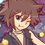# Inequalities (Thailand Math POSN 1st elimination round 2014)

Only use any the following.

• Basic inequalities
• AM-GM-HM
• Holder
• Cauchy Schwarz (Titu's lemma is acceptable)
• Weighted AM-GM

1.) Let $$a,b,c$$ be positive real numbers such that $$abc = 1$$. Prove that

$\frac{4}{(a+2)^{2}+4b^{2}} + \frac{4}{(b+2)^{2}+4c^{2}} + \frac{4}{(c+2)^{2}+4a^{2}} \leq 1$

2.) Find all positive real solutions $a,b,c$ such that

$\begin{cases} 10a^{3}+b^{3} = 7ab + 20bc - 11 \\ b^{3}+20c^{3} = 7bc + 30ca - 20 \\ 34c^{3}+44a^{3} = 51ca + 20ab - 50 \\ \end{cases}$

3.) Let $a,b,c$ be positive real numbers such that $abc = 1$. Prove that

$a^{3}b+b^{3}c+c^{3}a \geq a^{2/5}b^{3/5} + b^{2/5}c^{3/5} + c^{2/5}a^{3/5}$

4.) Let $a,b,c$ be positive real numbers such that $a+b+c+abc = 4$. Prove that

$\left(\frac{a}{\sqrt{b+c}}+\frac{b}{\sqrt{c+a}}+\frac{c}{\sqrt{a+b}}\right)^{2}(ab+bc+ca) \geq \frac{1}{2}(4-abc)^{3}$

5.) Let $a,b,c$ be positive real numbers. Prove that

$\frac{a^{9}}{bc}+\frac{b^{9}}{ca}+\frac{c^{9}}{ab} + \frac{3}{abc} \geq a^{4}+b^{4}+c^{4}+3$

Check out all my notes and stuffs for more problems!

Thailand Math POSN 2013

Thailand Math POSN 2014Note by Samuraiwarm Tsunayoshi
6 years, 7 months ago

This discussion board is a place to discuss our Daily Challenges and the math and science related to those challenges. Explanations are more than just a solution — they should explain the steps and thinking strategies that you used to obtain the solution. Comments should further the discussion of math and science.

When posting on Brilliant:

• Use the emojis to react to an explanation, whether you're congratulating a job well done , or just really confused .
• Ask specific questions about the challenge or the steps in somebody's explanation. Well-posed questions can add a lot to the discussion, but posting "I don't understand!" doesn't help anyone.
• Try to contribute something new to the discussion, whether it is an extension, generalization or other idea related to the challenge.
• Stay on topic — we're all here to learn more about math and science, not to hear about your favorite get-rich-quick scheme or current world events.

MarkdownAppears as
*italics* or _italics_ italics
**bold** or __bold__ bold
- bulleted- list
• bulleted
• list
1. numbered2. list
1. numbered
2. list
Note: you must add a full line of space before and after lists for them to show up correctly
paragraph 1paragraph 2

paragraph 1

paragraph 2

[example link](https://brilliant.org)example link
> This is a quote
This is a quote
    # I indented these lines
# 4 spaces, and now they show
# up as a code block.

print "hello world"
# I indented these lines
# 4 spaces, and now they show
# up as a code block.

print "hello world"
MathAppears as
Remember to wrap math in $$ ... $$ or $ ... $ to ensure proper formatting.
2 \times 3 $2 \times 3$
2^{34} $2^{34}$
a_{i-1} $a_{i-1}$
\frac{2}{3} $\frac{2}{3}$
\sqrt{2} $\sqrt{2}$
\sum_{i=1}^3 $\sum_{i=1}^3$
\sin \theta $\sin \theta$
\boxed{123} $\boxed{123}$

## Comments

Sort by:

Top Newest

I like the kind of questions where a suitably application of AM-GM gives the resultant inequality, since that relies on your ability to spot / identify patterns.

For example, here is how to do 3:

Apply AM-GM to $2 a^3 b + 2 b^3 c + c^3 a$.

Staff - 6 years, 7 months ago

Log in to reply

Multiply $abc$ on the RHS. Then Muirhead.

Muirheaders gonna Muirhead Muirhead Muirhead

- 6 years, 7 months ago

Log in to reply

Right. Muirhead is just a fancy term for bunching AM-GM.

Staff - 6 years, 7 months ago

Log in to reply

In addition, graders hate it because it involves no ingenuity at all.

- 6 years, 7 months ago

Log in to reply

Sorry I miss typed no.4 here. It is $a+b+c+abc = 4$ instead of $1$.

- 6 years, 7 months ago

Log in to reply

Is it necessary to use just the ineqs u stated?

- 6 years, 7 months ago

Log in to reply

Yup.

- 6 years, 7 months ago

Log in to reply

×

Problem Loading...

Note Loading...

Set Loading...